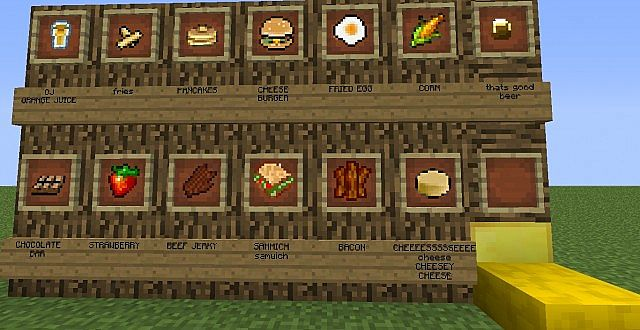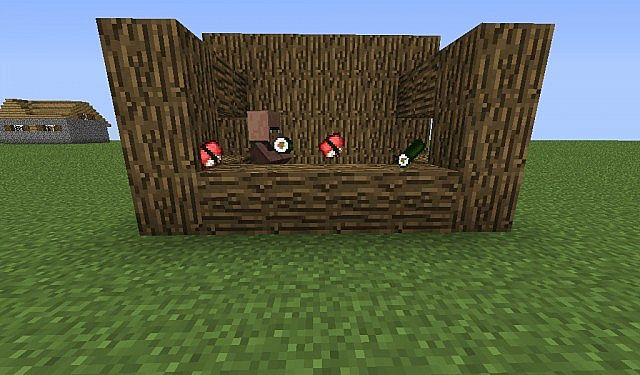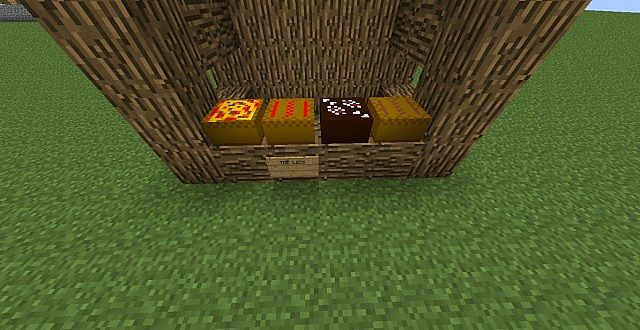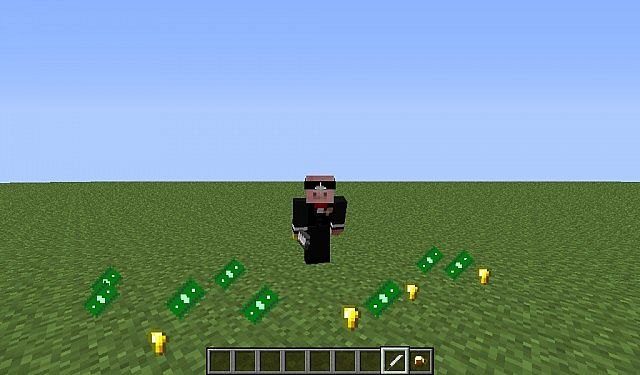• 2

posted a message on [1.6.4] [FORGE] Mo' food and crops.
Hey guys, this is an update to a mod originally by v3XzZ. I was on the mod dev team for it, and he has become inactive for some time now, so I asked around and got some help updating this to 1.6.4, I'll be updating from here on with help from master801.

If and when v3XzZ comes back, and he asks me to, I'll take this down.

Original topic: http://www.minecraft...-showing-items/https://www.dropbox.....4)-mFC-SRG.zip

install forge 9.11.1.965 for minecraft 1.6.4,
http://files.minecraftforge.net/

Drag this mod into your .minecraft/mods folder,

old:

If you have bugs let me know

Screenshots:

Some of the foodsSushi!The lies...Money!Videos

[media][media][/media[/media]]

Credits:

Leanardoe - Current mod developer
master801 - Source code and update to 1.6.4 help
v3XzZ - Original mod developer
Lordplantitas - for awesome texture sprites
J0hnnzZ - for some older texture sprites and ideas and a lot of help
OriginalElfkin - for a HD texture(not done yet)
Bloo1234 and Honeybee99 - for lot of work on the wiki

Plans:

Update to 1.7.X
Revision of a lot of textures.
Fix all sound bugs
Modify drop rates
Fix not having a height limit on bugs
Remove redundant features
Maybe - integrate with other mods

(Bare with me guys I'm new to modding, but then again I'm not new to java so we'll see.)

Oh and check out the wiki!
http://mofc.wikia.co..._and_Crops_Wiki
Posted in: Minecraft Mods
• 1

posted a message on Ban the user above you #2
Quote from engie_ninja

Banned for a reason that I feel uncomfortable explaining.

Banned because killing floor is fun
Quote from BloodyPhoenix

Banned until someone fixes the n's.

Banned because you posted before me
Posted in: Forum Games
• 1

Quote from Frostbreath

I would very much like to hear something from the mod author about the status. It has been over a week since he last posted. What happened man?

He is working very hard to code in peace.
Posted in: Minecraft Mods
• 1

posted a message on Mo' Creatures - v12.0.0 for Minecraft 1.12.1!! Now Opensource!!
Quote from ihsan07

Same here. Hope it will be fixed with new update

on ssp, but not on smp, and skeletons don't have bows.

Quote from PYROxSYCO

i think your mod messed with the skeletons they act like zombies and naturally don't spawn with bows unless spawned with a spawn egg but still have the same behavior as zombies

same here, but they are fast.
Posted in: Minecraft Mods
• 1

posted a message on Help when i look at mob i crashed
Quote from ds730

Hello Im back to mod again and when i look at my mob i crash everythings fine heres my error
---- Minecraft Crash Report ----
// Shall we play a game?

Time: 12/22/12 5:30 PM
Description: Exception in world tick

java.lang.NullPointerException
at net.minecraft.src.NetClientHandler.handleMobSpawn(NetClientHandler.java:753)
at net.minecraft.src.Packet24MobSpawn.processPacket(Packet24MobSpawn.java:137)
at net.minecraft.src.WorldClient.tick(WorldClient.java:64)
at net.minecraft.client.Minecraft.runTick(Minecraft.java:1861)
at net.minecraft.client.Minecraft.runGameLoop(Minecraft.java:843)
at net.minecraft.client.Minecraft.run(Minecraft.java:768)

A detailed walkthrough of the error, its code path and all known details is as follows:
---------------------------------------------------------------------------------------

Stacktrace:
at net.minecraft.src.NetClientHandler.handleMobSpawn(NetClientHandler.java:753)
at net.minecraft.src.Packet24MobSpawn.processPacket(Packet24MobSpawn.java:137)

-- Affected level --
Details:
Level name: MpServer
All players: 1 total; [EntityClientPlayerMP['Player78'/456, l='MpServer', x=-237.03, y=69.62, z=173.97]]
Chunk stats: MultiplayerChunkCache: 441
Level seed: 0
Level generator: ID 00 - default, ver 1. Features enabled: false
Level generator options:
Level spawn location: World: (-208,64,256), Chunk: (at 0,4,0 in -13,16; contains blocks -208,0,256 to -193,255,271), Region: (-1,0; contains chunks -32,0 to -1,31, blocks -512,0,0 to -1,255,511)
Level time: 1030 game time, 1030 day time
Level dimension: 0
Level storage version: 0x00000 - Unknown?
Level weather: Rain time: 0 (now: false), thunder time: 0 (now: false)
Level game mode: Game mode: creative (ID 1). Hardcore: false. Cheats: false
Forced entities: 168 total; [EntityPig['Pig'/275, l='MpServer', x=-199.02, y=73.00, z=123.93], EntityPig['Pig'/274, l='MpServer', x=-200.34, y=72.00, z=117.53], EntityChicken['Chicken'/279, l='MpServer', x=-197.50, y=65.00, z=140.50], EntityChicken['Chicken'/278, l='MpServer', x=-199.50, y=63.00, z=143.50], EntityPig['Pig'/277, l='MpServer', x=-201.69, y=72.00, z=116.75], EntityPig['Pig'/276, l='MpServer', x=-201.04, y=72.00, z=111.20], EntityClientPlayerMP['Player78'/456, l='MpServer', x=-237.03, y=69.62, z=173.97], EntityChicken['Chicken'/283, l='MpServer', x=-199.50, y=63.00, z=146.50], EntityPig['Pig'/282, l='MpServer', x=-193.34, y=63.00, z=151.47], EntityPig['Pig'/281, l='MpServer', x=-193.50, y=71.00, z=140.19], EntityChicken['Chicken'/280, l='MpServer', x=-195.47, y=69.00, z=141.38], EntityWolf['Wolf'/287, l='MpServer', x=-207.41, y=77.00, z=215.88], EntityCreeper['Creeper'/286, l='MpServer', x=-192.94, y=16.00, z=197.66], EntityBat['Bat'/285, l='MpServer', x=-193.75, y=14.10, z=185.34], EntityPig['Pig'/284, l='MpServer', x=-198.91, y=64.00, z=153.13], EntityWolf['Wolf'/258, l='MpServer', x=-209.72, y=77.00, z=202.50], EntityWolf['Wolf'/259, l='MpServer', x=-208.50, y=77.00, z=213.50], EntitySpider['Spider'/256, l='MpServer', x=-208.94, y=58.00, z=194.97], EntityWolf['Wolf'/257, l='MpServer', x=-216.59, y=77.00, z=202.69], EntityWolf['Wolf'/262, l='MpServer', x=-211.50, y=79.00, z=232.50], EntityWolf['Wolf'/263, l='MpServer', x=-200.50, y=78.00, z=224.41], EntityWolf['Wolf'/260, l='MpServer', x=-215.25, y=78.00, z=222.41], EntityWolf['Wolf'/261, l='MpServer', x=-217.56, y=84.00, z=215.09], EntityPig['Pig'/264, l='MpServer', x=-208.03, y=66.00, z=240.38], EntityPig['Pig'/265, l='MpServer', x=-205.59, y=66.00, z=243.88], EntityFallingSand['Falling Block'/29, l='MpServer', x=-349.50, y=38.86, z=229.50], EntityFallingSand['Falling Block'/31, l='MpServer', x=-351.50, y=38.86, z=232.50], EntityFallingSand['Falling Block'/30, l='MpServer', x=-350.50, y=38.86, z=229.50], EntityPig['Pig'/304, l='MpServer', x=-203.72, y=66.00, z=244.88], EntityFallingSand['Falling Block'/39, l='MpServer', x=-350.50, y=38.86, z=242.50], EntityCreeper['Creeper'/313, l='MpServer', x=-186.50, y=12.00, z=199.50], EntityPig['Pig'/312, l='MpServer', x=-191.50, y=63.00, z=150.50], EntityFallingSand['Falling Block'/40, l='MpServer', x=-351.50, y=38.86, z=242.50], EntitySkeleton['Skeleton'/315, l='MpServer', x=-184.50, y=13.00, z=190.16], EntityFallingSand['Falling Block'/41, l='MpServer', x=-351.50, y=38.86, z=243.50], EntitySkeleton['Skeleton'/314, l='MpServer', x=-182.41, y=14.00, z=194.84], EntityZombie['Zombie'/317, l='MpServer', x=-188.50, y=58.00, z=208.50], EntityBat['Bat'/316, l='MpServer', x=-181.25, y=19.43, z=221.50], EntityWolf['Wolf'/319, l='MpServer', x=-189.50, y=77.00, z=214.50], EntityWolf['Wolf'/318, l='MpServer', x=-189.50, y=77.00, z=213.50], EntityWolf['Wolf'/288, l='MpServer', x=-203.90, y=83.00, z=218.78], EntityWolf['Wolf'/289, l='MpServer', x=-206.50, y=77.00, z=218.50], EntityWolf['Wolf'/290, l='MpServer', x=-206.50, y=77.00, z=217.50], EntityWolf['Wolf'/291, l='MpServer', x=-203.47, y=82.00, z=214.81], EntityWolf['Wolf'/292, l='MpServer', x=-192.50, y=78.00, z=211.50], EntityPig['Pig'/293, l='MpServer', x=-201.34, y=70.00, z=227.47], EntityPig['Pig'/294, l='MpServer', x=-202.09, y=67.00, z=231.94], EntityPig['Pig'/295, l='MpServer', x=-201.50, y=69.00, z=227.50], EntityPig['Pig'/296, l='MpServer', x=-201.59, y=68.00, z=228.47], EntityWolf['Wolf'/297, l='MpServer', x=-204.50, y=68.00, z=231.01], EntityWolf['Wolf'/298, l='MpServer', x=-197.47, y=71.93, z=225.69], EntityPig['Pig'/299, l='MpServer', x=-206.19, y=67.00, z=233.47], EntityWolf['Wolf'/300, l='MpServer', x=-197.50, y=67.00, z=234.50], EntityWolf['Wolf'/301, l='MpServer', x=-196.53, y=68.00, z=232.21], EntityWolf['Wolf'/302, l='MpServer', x=-193.50, y=73.00, z=227.80], EntityWolf['Wolf'/303, l='MpServer', x=-201.51, y=72.00, z=226.85], EntitySkeleton['Skeleton'/341, l='MpServer', x=-169.50, y=40.00, z=227.50], EntityItem['item.tile.sapling.oak'/340, l='MpServer', x=-173.47, y=81.13, z=178.16], EntityWolf['Wolf'/339, l='MpServer', x=-166.50, y=68.00, z=184.50], EntityWolf['Wolf'/338, l='MpServer', x=-167.50, y=68.00, z=185.50], EntityWolf['Wolf'/337, l='MpServer', x=-165.50, y=68.00, z=185.50], EntityWolf['Wolf'/336, l='MpServer', x=-166.50, y=68.00, z=187.50], EntitySkeleton['Skeleton'/77, l='MpServer', x=-315.50, y=60.00, z=155.50], EntityCreeper['Creeper'/78, l='MpServer', x=-310.50, y=60.00, z=153.50], EntityWolf['Wolf'/79, l='MpServer', x=-307.50, y=75.00, z=147.50], EntityZombie['Zombie'/326, l='MpServer', x=-184.53, y=48.00, z=241.96], EntitySpider['Spider'/324, l='MpServer', x=-179.19, y=50.00, z=249.28], EntityZombie['Zombie'/325, l='MpServer', x=-179.69, y=49.00, z=252.44], EntityWolf['Wolf'/81, l='MpServer', x=-310.50, y=65.00, z=150.50], EntitySpider['Spider'/322, l='MpServer', x=-187.94, y=57.00, z=226.28], EntityWolf['Wolf'/80, l='MpServer', x=-308.50, y=75.00, z=147.50], EntityBat['Bat'/323, l='MpServer', x=-183.66, y=18.56, z=231.75], EntityWolf['Wolf'/320, l='MpServer', x=-188.50, y=77.00, z=213.50], EntityWolf['Wolf'/82, l='MpServer', x=-307.50, y=66.00, z=150.50], EntitySkeleton['Skeleton'/321, l='MpServer', x=-191.50, y=55.00, z=231.50], EntityBat['Bat'/92, l='MpServer', x=-304.53, y=33.21, z=236.09], EntityPig['Pig'/335, l='MpServer', x=-160.50, y=72.00, z=154.25], EntityCreeper['Creeper'/95, l='MpServer', x=-311.50, y=32.00, z=245.25], EntityPig['Pig'/332, l='MpServer', x=-162.50, y=70.00, z=121.50], EntityPig['Pig'/333, l='MpServer', x=-167.95, y=71.00, z=116.02], EntityWolf['Wolf'/89, l='MpServer', x=-311.22, y=64.00, z=175.38], EntityPig['Pig'/330, l='MpServer', x=-164.69, y=70.00, z=126.50], EntityWolf['Wolf'/88, l='MpServer', x=-315.47, y=71.00, z=170.55], EntityPig['Pig'/331, l='MpServer', x=-160.50, y=70.00, z=121.47], EntityCreeper['Creeper'/91, l='MpServer', x=-304.78, y=33.00, z=236.01], EntityBat['Bat'/329, l='MpServer', x=-162.38, y=55.82, z=116.31], EntityWolf['Wolf'/90, l='MpServer', x=-306.50, y=65.00, z=176.50], EntityWolf['Wolf'/110, l='MpServer', x=-289.69, y=66.00, z=103.25], EntityBat['Bat'/111, l='MpServer', x=-288.56, y=15.10, z=109.72], EntityItem['item.tile.sapling.oak'/115, l='MpServer', x=-296.94, y=73.13, z=224.50], EntityWolf['Wolf'/114, l='MpServer', x=-302.50, y=64.00, z=184.16], EntityItem['item.tile.sapling.oak'/113, l='MpServer', x=-300.31, y=79.13, z=117.22], EntityItem['item.item.apple'/112, l='MpServer', x=-298.88, y=77.13, z=126.69], EntitySpider['Spider'/127, l='MpServer', x=-278.22, y=46.00, z=100.38], EntityWolf['Wolf'/136, l='MpServer', x=-282.50, y=66.00, z=192.50], EntityWolf['Wolf'/139, l='MpServer', x=-260.44, y=72.00, z=94.69], EntityWolf['Wolf'/138, l='MpServer', x=-258.69, y=71.00, z=95.69], EntityWolf['Wolf'/141, l='MpServer', x=-270.31, y=70.00, z=141.09], EntityWolf['Wolf'/140, l='MpServer', x=-278.31, y=69.00, z=137.90], EntityPig['Pig'/143, l='MpServer', x=-269.97, y=68.00, z=133.09], EntityWolf['Wolf'/142, l='MpServer', x=-270.34, y=70.00, z=141.97], EntityItem['item.tile.sapling.oak'/129, l='MpServer', x=-272.75, y=78.13, z=118.84], EntityItem['item.tile.sapling.oak'/128, l='MpServer', x=-277.59, y=78.13, z=124.53], EntityPig['Pig'/131, l='MpServer', x=-272.53, y=70.00, z=141.53], EntityWolf['Wolf'/130, l='MpServer', x=-273.00, y=70.00, z=140.28], EntityWolf['Wolf'/133, l='MpServer', x=-283.50, y=66.00, z=191.50], EntityCreeper['Creeper'/132, l='MpServer', x=-286.53, y=20.00, z=163.97], EntityWolf['Wolf'/135, l='MpServer', x=-281.50, y=66.00, z=192.50], EntityWolf['Wolf'/134, l='MpServer', x=-284.59, y=66.00, z=189.22], EntitySkeleton['Skeleton'/152, l='MpServer', x=-259.01, y=50.00, z=159.50], EntitySpider['Spider'/153, l='MpServer', x=-257.53, y=56.00, z=190.49], EntitySkeleton['Skeleton'/154, l='MpServer', x=-265.50, y=18.00, z=217.50], EntityEnderman['Enderman'/155, l='MpServer', x=-266.63, y=21.00, z=239.09], EntitySkeleton['Skeleton'/156, l='MpServer', x=-264.69, y=16.00, z=250.50], EntityEnderman['Enderman'/157, l='MpServer', x=-266.50, y=21.00, z=240.50], EntitySkeleton['Skeleton'/158, l='MpServer', x=-253.59, y=57.00, z=251.59], EntityPig['Pig'/144, l='MpServer', x=-267.69, y=69.00, z=134.97], EntityPig['Pig'/145, l='MpServer', x=-277.82, y=68.00, z=132.19], EntityItem['item.tile.sapling.oak'/146, l='MpServer', x=-268.06, y=77.13, z=133.88], EntityItem['item.tile.sapling.oak'/147, l='MpServer', x=-257.38, y=81.13, z=132.88], EntitySkeleton['Skeleton'/148, l='MpServer', x=-263.50, y=50.00, z=157.50], EntitySkeleton['Skeleton'/149, l='MpServer', x=-264.50, y=50.00, z=158.50], EntitySpider['Spider'/150, l='MpServer', x=-261.75, y=61.00, z=174.31], EntitySkeleton['Skeleton'/151, l='MpServer', x=-267.59, y=51.00, z=160.97], EntityItem['item.item.apple'/1524, l='MpServer', x=-273.63, y=79.13, z=117.62], EntityItem['item.tile.sapling.oak'/1531, l='MpServer', x=-279.13, y=80.13, z=118.25], EntityWolf['Wolf'/186, l='MpServer', x=-248.13, y=70.00, z=98.47], EntitySkeleton['Skeleton'/187, l='MpServer', x=-244.50, y=35.00, z=117.03], EntityWolf['Wolf'/185, l='MpServer', x=-255.69, y=70.00, z=98.38], EntityItem['item.tile.gravel'/190, l='MpServer', x=-244.03, y=31.13, z=161.97], EntityItem['item.tile.gravel'/191, l='MpServer', x=-245.63, y=33.13, z=162.88], EntitySkeleton['Skeleton'/188, l='MpServer', x=-258.75, y=20.00, z=154.99], EntitySkeleton['Skeleton'/189, l='MpServer', x=-254.03, y=18.00, z=155.31], EntityCreeper['Creeper'/196, l='MpServer', x=-252.50, y=19.00, z=200.50], EntityCreeper['Creeper'/193, l='MpServer', x=-252.50, y=39.00, z=164.50], EntityItem['item.tile.gravel'/192, l='MpServer', x=-246.88, y=34.13, z=162.16], EntityCreeper['Creeper'/195, l='MpServer', x=-250.50, y=19.00, z=200.50], EntityCreeper['Creeper'/194, l='MpServer', x=-240.50, y=34.00, z=170.50], EntityPig['Pig'/220, l='MpServer', x=-235.94, y=65.00, z=95.88], EntityPig['Pig'/221, l='MpServer', x=-230.72
, y=64.00, z=96.47], EntitySkeleton['Skeleton'/222, l='MpServer', x=-232.78, y=48.00, z=112.16], EntitySkeleton['Skeleton'/223, l='MpServer', x=-227.50, y=45.00, z=115.50], EntityPig['Pig'/219, l='MpServer', x=-231.35, y=63.00, z=98.84], EntityBat['Bat'/213, l='MpServer', x=-223.94, y=35.99, z=101.06], EntityZombie['Zombie'/239, l='MpServer', x=-234.50, y=24.00, z=246.50], EntityEnderman['Enderman'/238, l='MpServer', x=-233.28, y=18.00, z=239.69], EntityEnderman['Enderman'/237, l='MpServer', x=-234.50, y=17.00, z=238.50], EntityWolf['Wolf'/236, l='MpServer', x=-232.78, y=80.00, z=221.47], EntityWolf['Wolf'/235, l='MpServer', x=-234.50, y=79.00, z=194.00], EntityBat['Bat'/234, l='MpServer', x=-227.53, y=41.10, z=183.22], EntityCreeper['Creeper'/233, l='MpServer', x=-225.59, y=51.00, z=163.09], EntityCreeper['Creeper'/232, l='MpServer', x=-234.50, y=34.00, z=171.50], EntityEnderman['Enderman'/231, l='MpServer', x=-236.22, y=33.00, z=169.75], EntityZombie['Zombie'/230, l='MpServer', x=-239.44, y=33.00, z=173.04], EntityZombie['Zombie'/229, l='MpServer', x=-231.28, y=12.00, z=156.34], EntitySkeleton['Skeleton'/228, l='MpServer', x=-239.87, y=52.00, z=155.38], EntityEnderman['Enderman'/227, l='MpServer', x=-231.50, y=35.00, z=153.50], EntitySkeleton['Skeleton'/226, l='MpServer', x=-236.56, y=26.00, z=152.75], EntityZombie['Zombie'/225, l='MpServer', x=-229.50, y=50.00, z=139.50], EntitySkeleton['Skeleton'/224, l='MpServer', x=-224.50, y=45.00, z=117.50], EntityCreeper['Creeper'/254, l='MpServer', x=-223.63, y=51.00, z=162.22], EntityBat['Bat'/255, l='MpServer', x=-220.34, y=42.00, z=204.66], EntityCreeper['Creeper'/252, l='MpServer', x=-223.50, y=27.00, z=137.50], EntityCreeper['Creeper'/253, l='MpServer', x=-221.50, y=51.00, z=164.50], EntityItem['item.item.seeds'/250, l='MpServer', x=-218.06, y=63.13, z=115.66], EntitySpider['Spider'/251, l='MpServer', x=-214.91, y=27.00, z=138.38], EntityBat['Bat'/249, l='MpServer', x=-216.38, y=37.10, z=107.97], EntityZombie['Zombie'/240, l='MpServer', x=-236.50, y=24.00, z=243.50]]
Retry entities: 0 total; []
Stacktrace:
at net.minecraft.client.Minecraft.runTick(Minecraft.java:1874)
at net.minecraft.client.Minecraft.runGameLoop(Minecraft.java:843)
at net.minecraft.client.Minecraft.run(Minecraft.java:768)

-- Affected level --
Details:
Level name: MpServer
All players: 1 total; [EntityClientPlayerMP['Player78'/456, l='MpServer', x=-237.03, y=69.62, z=173.97]]
Chunk stats: MultiplayerChunkCache: 441
Level seed: 0
Level generator: ID 00 - default, ver 1. Features enabled: false
Level generator options:
Level spawn location: World: (-208,64,256), Chunk: (at 0,4,0 in -13,16; contains blocks -208,0,256 to -193,255,271), Region: (-1,0; contains chunks -32,0 to -1,31, blocks -512,0,0 to -1,255,511)
Level time: 1030 game time, 1030 day time
Level dimension: 0
Level storage version: 0x00000 - Unknown?
Level weather: Rain time: 0 (now: false), thunder time: 0 (now: false)
Level game mode: Game mode: creative (ID 1). Hardcore: false. Cheats: false
Forced entities: 168 total; [EntityPig['Pig'/275, l='MpServer', x=-199.02, y=73.00, z=123.93], EntityPig['Pig'/274, l='MpServer', x=-200.34, y=72.00, z=117.53], EntityChicken['Chicken'/279, l='MpServer', x=-197.50, y=65.00, z=140.50], EntityChicken['Chicken'/278, l='MpServer', x=-199.50, y=63.00, z=143.50], EntityPig['Pig'/277, l='MpServer', x=-201.69, y=72.00, z=116.75], EntityPig['Pig'/276, l='MpServer', x=-201.04, y=72.00, z=111.20], EntityClientPlayerMP['Player78'/456, l='MpServer', x=-237.03, y=69.62, z=173.97], EntityChicken['Chicken'/283, l='MpServer', x=-199.50, y=63.00, z=146.50], EntityPig['Pig'/282, l='MpServer', x=-193.34, y=63.00, z=151.47], EntityPig['Pig'/281, l='MpServer', x=-193.50, y=71.00, z=140.19], EntityChicken['Chicken'/280, l='MpServer', x=-195.47, y=69.00, z=141.38], EntityWolf['Wolf'/287, l='MpServer', x=-207.41, y=77.00, z=215.88], EntityCreeper['Creeper'/286, l='MpServer', x=-192.94, y=16.00, z=197.66], EntityBat['Bat'/285, l='MpServer', x=-193.75, y=14.10, z=185.34], EntityPig['Pig'/284, l='MpServer', x=-198.91, y=64.00, z=153.13], EntityWolf['Wolf'/258, l='MpServer', x=-209.72, y=77.00, z=202.50], EntityWolf['Wolf'/259, l='MpServer', x=-208.50, y=77.00, z=213.50], EntitySpider['Spider'/256, l='MpServer', x=-208.94, y=58.00, z=194.97], EntityWolf['Wolf'/257, l='MpServer', x=-216.59, y=77.00, z=202.69], EntityWolf['Wolf'/262, l='MpServer', x=-211.50, y=79.00, z=232.50], EntityWolf['Wolf'/263, l='MpServer', x=-200.50, y=78.00, z=224.41], EntityWolf['Wolf'/260, l='MpServer', x=-215.25, y=78.00, z=222.41], EntityWolf['Wolf'/261, l='MpServer', x=-217.56, y=84.00, z=215.09], EntityPig['Pig'/264, l='MpServer', x=-208.03, y=66.00, z=240.38], EntityPig['Pig'/265, l='MpServer', x=-205.59, y=66.00, z=243.88], EntityFallingSand['Falling Block'/29, l='MpServer', x=-349.50, y=38.86, z=229.50], EntityFallingSand['Falling Block'/31, l='MpServer', x=-351.50, y=38.86, z=232.50], EntityFallingSand['Falling Block'/30, l='MpServer', x=-350.50, y=38.86, z=229.50], EntityPig['Pig'/304, l='MpServer', x=-203.72, y=66.00, z=244.88], EntityFallingSand['Falling Block'/39, l='MpServer', x=-350.50, y=38.86, z=242.50], EntityCreeper['Creeper'/313, l='MpServer', x=-186.50, y=12.00, z=199.50], EntityPig['Pig'/312, l='MpServer', x=-191.50, y=63.00, z=150.50], EntityFallingSand['Falling Block'/40, l='MpServer', x=-351.50, y=38.86, z=242.50], EntitySkeleton['Skeleton'/315, l='MpServer', x=-184.50, y=13.00, z=190.16], EntityFallingSand['Falling Block'/41, l='MpServer', x=-351.50, y=38.86, z=243.50], EntitySkeleton['Skeleton'/314, l='MpServer', x=-182.41, y=14.00, z=194.84], EntityZombie['Zombie'/317, l='MpServer', x=-188.50, y=58.00, z=208.50], EntityBat['Bat'/316, l='MpServer', x=-181.25, y=19.43, z=221.50], EntityWolf['Wolf'/319, l='MpServer', x=-189.50, y=77.00, z=214.50], EntityWolf['Wolf'/318, l='MpServer', x=-189.50, y=77.00, z=213.50], EntityWolf['Wolf'/288, l='MpServer', x=-203.90, y=83.00, z=218.78], EntityWolf['Wolf'/289, l='MpServer', x=-206.50, y=77.00, z=218.50], EntityWolf['Wolf'/290, l='MpServer', x=-206.50, y=77.00, z=217.50], EntityWolf['Wolf'/291, l='MpServer', x=-203.47, y=82.00, z=214.81], EntityWolf['Wolf'/292, l='MpServer', x=-192.50, y=78.00, z=211.50], EntityPig['Pig'/293, l='MpServer', x=-201.34, y=70.00, z=227.47], EntityPig['Pig'/294, l='MpServer', x=-202.09, y=67.00, z=231.94], EntityPig['Pig'/295, l='MpServer', x=-201.50, y=69.00, z=227.50], EntityPig['Pig'/296, l='MpServer', x=-201.59, y=68.00, z=228.47], EntityWolf['Wolf'/297, l='MpServer', x=-204.50, y=68.00, z=231.01], EntityWolf['Wolf'/298, l='MpServer', x=-197.47, y=71.93, z=225.69], EntityPig['Pig'/299, l='MpServer', x=-206.19, y=67.00, z=233.47], EntityWolf['Wolf'/300, l='MpServer', x=-197.50, y=67.00, z=234.50], EntityWolf['Wolf'/301, l='MpServer', x=-196.53, y=68.00, z=232.21], EntityWolf['Wolf'/302, l='MpServer', x=-193.50, y=73.00, z=227.80], EntityWolf['Wolf'/303, l='MpServer', x=-201.51, y=72.00, z=226.85], EntitySkeleton['Skeleton'/341, l='MpServer', x=-169.50, y=40.00, z=227.50], EntityItem['item.tile.sapling.oak'/340, l='MpServer', x=-173.47, y=81.13, z=178.16], EntityWolf['Wolf'/339, l='MpServer', x=-166.50, y=68.00, z=184.50], EntityWolf['Wolf'/338, l='MpServer', x=-167.50, y=68.00, z=185.50], EntityWolf['Wolf'/337, l='MpServer', x=-165.50, y=68.00, z=185.50], EntityWolf['Wolf'/336, l='MpServer', x=-166.50, y=68.00, z=187.50], EntitySkeleton['Skeleton'/77, l='MpServer', x=-315.50, y=60.00, z=155.50], EntityCreeper['Creeper'/78, l='MpServer', x=-310.50, y=60.00, z=153.50], EntityWolf['Wolf'/79, l='MpServer', x=-307.50, y=75.00, z=147.50], EntityZombie['Zombie'/326, l='MpServer', x=-184.53, y=48.00, z=241.96], EntitySpider['Spider'/324, l='MpServer', x=-179.19, y=50.00, z=249.28], EntityZombie['Zombie'/325, l='MpServer', x=-179.69, y=49.00, z=252.44], EntityWolf['Wolf'/81, l='MpServer', x=-310.50, y=65.00, z=150.50], EntitySpider['Spider'/322, l='MpServer', x=-187.94, y=57.00, z=226.28], EntityWolf['Wolf'/80, l='MpServer', x=-308.50, y=75.00, z=147.50], EntityBat['Bat'/323, l='MpServer', x=-183.66, y=18.56, z=231.75], EntityWolf['Wolf'/320, l='MpServer', x=-188.50, y=77.00, z=213.50], EntityWolf['Wolf'/82, l='MpServer', x=-307.50, y=66.00, z=150.50], EntitySkeleton['Skeleton'/321, l='MpServer', x=-191.50, y=55.00, z=231.50], EntityBat['Bat'/92, l='MpServer', x=-304.53, y=33.21, z=236.09], EntityPig['Pig'/335, l='MpServer', x=-160.50, y=72.00, z=154.25], EntityCreeper['Creeper'/95, l='MpServer', x=-311.50, y=32.00, z=245.25], EntityPig['Pig'/332, l='MpServer', x=-162.50, y=70.00, z=121.50], EntityPig['Pig'/333, l='MpServer', x=-167.95, y=71.00, z=116.02], EntityWolf['Wolf'/89, l='MpServer', x=-311.22, y=64.00, z=175.38], EntityPig['Pig'/330, l='MpServer', x=-164.69, y=70.00, z=126.50], EntityWolf['Wolf'/88, l='MpServer', x=-315.47, y=71.00, z=170.55], EntityPig['Pig'/331, l='MpServer', x=-160.50, y=70.00, z=121.47], EntityCreeper['Creeper'/91, l='MpServer', x=-304.78, y=33.00, z=236.01], EntityBat['Bat'/329, l='MpServer', x=-162.38, y=55.82, z=116.31], EntityWolf['Wolf'/90, l='MpServer', x=-306.50, y=65.00, z=176.50], EntityWolf['Wolf'/110, l='MpServer', x=-289.69, y=66.00, z=103.25], EntityBat['Bat'/111, l='MpServer', x=-288.56, y=15.10, z=109.72], EntityItem['item.tile.sapling.oak'/115, l='MpServer', x=-296.94, y=73.13, z=224.50], EntityWolf['Wolf'/114, l='MpServer', x=-302.50, y=64.00, z=184.16], EntityItem['item.tile.sapling.oak'/113, l='MpServer', x=-300.31, y=79.13, z=117.22], EntityItem['item.item.apple'/112, l='MpServer', x=-298.88, y=77.13, z=126.69], EntitySpider['Spider'/127, l='MpServer', x=-278.22, y=46.00, z=100.38], EntityWolf['Wolf'/136, l='MpServer', x=-282.50, y=66.00, z=192.50], EntityWolf['Wolf'/139, l='MpServer', x=-260.44, y=72.00, z=94.69], EntityWolf['Wolf'/138, l='MpServer', x=-258.69, y=71.00, z=95.69], EntityWolf['Wolf'/141, l='MpServer', x=-270.31, y=70.00, z=141.09], EntityWolf['Wolf'/140, l='MpServer', x=-278.31, y=69.00, z=137.90], EntityPig['Pig'/143, l='MpServer', x=-269.97, y=68.00, z=133.09], EntityWolf['Wolf'/142, l='MpServer', x=-270.34, y=70.00, z=141.97], EntityItem['item.tile.sapling.oak'/129, l='MpServer', x=-272.75, y=78.13, z=118.84], EntityItem['item.tile.sapling.oak'/128, l='MpServer', x=-277.59, y=78.13, z=124.53], EntityPig['Pig'/131, l='MpServer', x=-272.53, y=70.00, z=141.53], EntityWolf['Wolf'/130, l='MpServer', x=-273.00, y=70.00, z=140.28], EntityWolf['Wolf'/133, l='MpServer', x=-283.50, y=66.00, z=191.50], EntityCreeper['Creeper'/132, l='MpServer', x=-286.53, y=20.00, z=163.97], EntityWolf['Wolf'/135, l='MpServer', x=-281.50, y=66.00, z=192.50], EntityWolf['Wolf'/134, l='MpServer', x=-284.59, y=66.00, z=189.22], EntitySkeleton['Skeleton'/152, l='MpServer', x=-259.01, y=50.00, z=159.50], EntitySpider['Spider'/153, l='MpServer', x=-257.53, y=56.00, z=190.49], EntitySkeleton['Skeleton'/154, l='MpServer', x=-265.50, y=18.00, z=217.50], EntityEnderman['Enderman'/155, l='MpServer', x=-266.63, y=21.00, z=239.09], EntitySkeleton['Skeleton'/156, l='MpServer', x=-264.69, y=16.00, z=250.50], EntityEnderman['Enderman'/157, l='MpServer', x=-266.50, y=21.00, z=240.50], EntitySkeleton['Skeleton'/158, l='MpServer', x=-253.59, y=57.00, z=251.59], EntityPig['Pig'/144, l='MpServer', x=-267.69, y=69.00, z=134.97], EntityPig['Pig'/145, l='MpServer', x=-277.82, y=68.00, z=132.19], EntityItem['item.tile.sapling.oak'/146, l='MpServer', x=-268.06, y=77.13, z=133.88], EntityItem['item.tile.sapling.oak'/147, l='MpServer', x=-257.38, y=81.13, z=132.88], EntitySkeleton['Skeleton'/148, l='MpServer', x=-263.50, y=50.00, z=157.50], EntitySkeleton['Skeleton'/149, l='MpServer', x=-264.50, y=50.00, z=158.50], EntitySpider['Spider'/150, l='MpServer', x=-261.75, y=61.00, z=174.31], EntitySkeleton['Skeleton'/151, l='MpServer', x=-267.59, y=51.00, z=160.97], EntityItem['item.item.apple'/1524, l='MpServer', x=-273.63, y=79.13, z=117.62], EntityItem['item.tile.sapling.oak'/1531, l='MpServer', x=-279.13, y=80.13, z=118.25], EntityWolf['Wolf'/186, l='MpServer', x=-248.13, y=70.00, z=98.47], EntitySkeleton['Skeleton'/187, l='MpServer', x=-244.50, y=35.00, z=117.03], EntityWolf['Wolf'/185, l='MpServer', x=-255.69, y=70.00, z=98.38], EntityItem['item.tile.gravel'/190, l='MpServer', x=-244.03, y=31.13, z=161.97], EntityItem['item.tile.gravel'/191, l='MpServer', x=-245.63, y=33.13, z=162.88], EntitySkeleton['Skeleton'/188, l='MpServer', x=-258.75, y=20.00, z=154.99], EntitySkeleton['Skeleton'/189, l='MpServer', x=-254.03, y=18.00, z=155.31], EntityCreeper['Creeper'/196, l='MpServer', x=-252.50, y=19.00, z=200.50], EntityCreeper['Creeper'/193, l='MpServer', x=-252.50, y=39.00, z=164.50], EntityItem['item.tile.gravel'/192, l='MpServer', x=-246.88, y=34.13, z=162.16], EntityCreeper['Creeper'/195, l='MpServer', x=-250.50, y=19.00, z=200.50], EntityCreeper['Creeper'/194, l='MpServer', x=-240.50, y=34.00, z=170.50], EntityPig['Pig'/220, l='MpServer', x=-235.94, y=65.00, z=95.88], EntityPig['Pig'/221, l='MpServer', x=-230.72
, y=64.00, z=96.47], EntitySkeleton['Skeleton'/222, l='MpServer', x=-232.78, y=48.00, z=112.16], EntitySkeleton['Skeleton'/223, l='MpServer', x=-227.50, y=45.00, z=115.50], EntityPig['Pig'/219, l='MpServer', x=-231.35, y=63.00, z=98.84], EntityBat['Bat'/213, l='MpServer', x=-223.94, y=35.99, z=101.06], EntityZombie['Zombie'/239, l='MpServer', x=-234.50, y=24.00, z=246.50], EntityEnderman['Enderman'/238, l='MpServer', x=-233.28, y=18.00, z=239.69], EntityEnderman['Enderman'/237, l='MpServer', x=-234.50, y=17.00, z=238.50], EntityWolf['Wolf'/236, l='MpServer', x=-232.78, y=80.00, z=221.47], EntityWolf['Wolf'/235, l='MpServer', x=-234.50, y=79.00, z=194.00], EntityBat['Bat'/234, l='MpServer', x=-227.53, y=41.10, z=183.22], EntityCreeper['Creeper'/233, l='MpServer', x=-225.59, y=51.00, z=163.09], EntityCreeper['Creeper'/232, l='MpServer', x=-234.50, y=34.00, z=171.50], EntityEnderman['Enderman'/231, l='MpServer', x=-236.22, y=33.00, z=169.75], EntityZombie['Zombie'/230, l='MpServer', x=-239.44, y=33.00, z=173.04], EntityZombie['Zombie'/229, l='MpServer', x=-231.28, y=12.00, z=156.34], EntitySkeleton['Skeleton'/228, l='MpServer', x=-239.87, y=52.00, z=155.38], EntityEnderman['Enderman'/227, l='MpServer', x=-231.50, y=35.00, z=153.50], EntitySkeleton['Skeleton'/226, l='MpServer', x=-236.56, y=26.00, z=152.75], EntityZombie['Zombie'/225, l='MpServer', x=-229.50, y=50.00, z=139.50], EntitySkeleton['Skeleton'/224, l='MpServer', x=-224.50, y=45.00, z=117.50], EntityCreeper['Creeper'/254, l='MpServer', x=-223.63, y=51.00, z=162.22], EntityBat['Bat'/255, l='MpServer', x=-220.34, y=42.00, z=204.66], EntityCreeper['Creeper'/252, l='MpServer', x=-223.50, y=27.00, z=137.50], EntityCreeper['Creeper'/253, l='MpServer', x=-221.50, y=51.00, z=164.50], EntityItem['item.item.seeds'/250, l='MpServer', x=-218.06, y=63.13, z=115.66], EntitySpider['Spider'/251, l='MpServer', x=-214.91, y=27.00, z=138.38], EntityBat['Bat'/249, l='MpServer', x=-216.38, y=37.10, z=107.97], EntityZombie['Zombie'/240, l='MpServer', x=-236.50, y=24.00, z=243.50]]
Retry entities: 0 total; []

-- System Details --
Details:
Minecraft Version: 1.4.6
Operating System: Windows Vista (x86) version 6.0
Java Version: 1.7.0_09, Oracle Corporation
Java VM Version: Java HotSpot™ Client VM (mixed mode), Oracle Corporation
Memory: 803788080 bytes (766 MB) / 1046937600 bytes (998 MB) up to 1046937600 bytes (998 MB)
JVM Flags: 3 total; -Xincgc -Xmx1024M -Xms1024M
AABB Pool Size: 12715 (712040 bytes; 0 MB) allocated, 2165 (121240 bytes; 0 MB) used
Suspicious classes: Start[net.minecraft.src.IPlayerUsage, MinecraftFakeLauncher, ThreadShutdown, ...]
IntCache: cache: 0, tcache: 0, allocated: 3, tallocated: 63
mod_Christmas 3.14159265

LWJGL: 2.4.2
OpenGL: ATI Radeon HD 4800 Series GL version 3.3.11399 Compatibility Profile Context, ATI Technologies Inc.
Is Modded: Very likely; Jar signature invalidated
Type: Client (map_client.txt)
Texture Pack: Default
Profiler Position: N/A (disabled)
Vec3 Pool Size: 504 (28224 bytes; 0 MB) allocated, 319 (17864 bytes; 0 MB) used

Looks like a render crash, Is your render code updated to the minecraft version you are useing? If so then use forge, it's API is more advanced than modloaders. I mean it has direct hooks into the mob render, thus supporting dynamic mob renders.
Posted in: Mods Discussion
• 1

posted a message on Mo Creatures Mod 1.4.6? How-To
Quote from Aidanny_Boy

Except from the official mod web site. i.e www.eloramm.com for redpower, or www.mod-buildcraft.com for buildrcraft.
Posted in: Mods Discussion
• 1

posted a message on [1.4.7] More Pistons
Quote from pauly21

Who are you to say "update this mod asap"???? why not do not update this mod, because of this kid.

There should be thumb down to give to users!!!

I guess the same reason you can say this. Being rude to someone just cause they are rude doesn't help anyone, just saying. just hit the report button instead.
Posted in: Minecraft Mods
• 1

posted a message on [1.3.2]The Fire Works Mod
Quote from caleb1011

You should try to make the firework mod for 360 version that would be cool!!

HAHAHAHA the xbox 360 version doesn't support mods
Posted in: Minecraft Mods
• 2

posted a message on Good Mods For Mod Showcases
i have 5,000...
-SCP CRAFT
-IC2
-MO CREATURES
- MO FOOD AND CROPS MOD
-ICBM
-nothing
-EXTRABIOMESXL
-SIMPLE GUNS MOD
-WEAPONS MOD
-CORAL REEF MOD
and
- THE FIRE WORKS MOD!!!!!!!!!!!!!!!!!!!!!!
Posted in: Mods Discussion
• 1

Quote from thin_doidle

dude. he is updating. modding takes a long time. its against the rules to ask for an update. just wait.

ic2 updated a month ago and its bigger than this mod
Posted in: Minecraft Mods